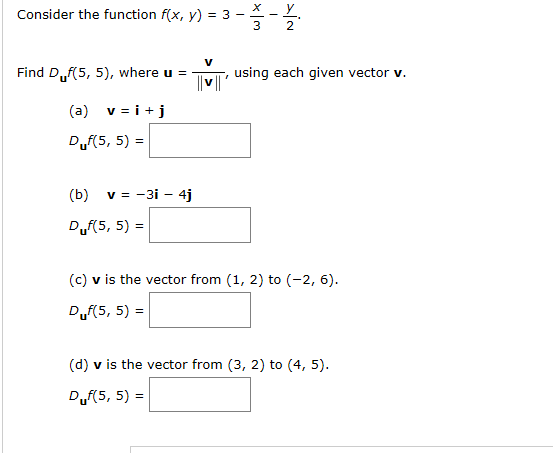# х _ уConsider the function f(x, y) = 3 -using each given vector v.Find D.,f(5, 5), where u =v i(a)Duf(5, 5) =v = -3i 4j(b)Duf(5, 5)(c) v is the vector from (1, 2) to (-2, 6)Duf(5, 5)(d) v is the vector from (3, 2) to (4, 5)Duf(5, 5)

Question
16 viewshelp_outlineImage Transcriptioncloseх _ у Consider the function f(x, y) = 3 - using each given vector v. Find D.,f(5, 5), where u = v i (a) Duf(5, 5) = v = -3i 4j (b) Duf(5, 5) (c) v is the vector from (1, 2) to (-2, 6) Duf(5, 5) (d) v is the vector from (3, 2) to (4, 5) Duf(5, 5) fullscreen
check_circle

Step 1

Since we only answer up to 3 sub-parts, we’ll answer the first 3. Please resubmit the question and specify the other subparts (up to 3) you’d like answered

Step 2

Given,

Step 3

Finding the directional derivative...

### Want to see the full answer?

See Solution

#### Want to see this answer and more?

Solutions are written by subject experts who are available 24/7. Questions are typically answered within 1 hour.*

See Solution
*Response times may vary by subject and question.
Tagged in

### Derivative